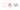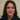# The CSS calc function explained with examples

Using the CSS calc function is great in many use cases. We will explain a few in this article with examples.

Generally the calc function is used to calculate CSS property values.  According to MDN we can use it anywhere a `<length>``<frequency>``<angle>``<time>``<percentage>``<number>`, or `<integer>` is allowed.

## Calc function syntax

Basically the below is its syntax with an example:

``````Syntax : property: calc(expression)
Example : width: calc(100% - 80px);``````

As a side note we need to remember a few things when using calc function:

• We can use  –  +  * /  math operators with it.
• When using  plus and minus sign we always should add a space between the sign and the number to make a valid parsable expression: calc(50% – 8px) is right. calc(50% -8px)  is wrong.
• We can use multiple units in our calc functions.### Positioning elements with calc

As an example we can position the background image in the bottom right using calc function. Look at this pen from CSS-tricks:

ttps://codepen.io/chriscoyier/pen/cqzmD

``````background-featuredImage: ../../static/images/design-with-code.jpg
# featuredImage: ../../static/images/url(dog.png);
background-position: calc(100% - 50px) calc(100% - 20px);``````

The above piece of code puts the dog background in the bottom right. As a result calc put it 50px from the right and 20px from the bottom of the window width.

### Calculate margins with calc

One of the best use cases we can use calc for is margins. Imagine we have a navigation on the left and an article tag on the right. Efficiently we can calculate the margin in between them and make room for both in the container using calc:

nav {
width: 20%;
margin-right: 1em;
float: left;
background: gold;
}
article {
width: calc(80% – 1em);
float: right;
background: orange;
}

We have a 1em margin to the right of the nav so in the article class we calculate that for article width so that they will not overlap each other. See the full example here.

### Calculate the height with calc

Generally we can use calc to calculate the height or make the content to have the full height needed. We have this CSS-tricks CodePen with header on the top and content in the bottom:

Therefor this is the interesting part of the code:

``````.area-one {
/* With the body as tall as the browser window
this will be too */
height: 100%;
}
.area-one h2 {
height: 50px;
line-height: 50px;
}
.content {
/* Subtract the header size */
height: calc(100% - 50px);
overflow: auto;
}``````

Firstly the body has a full height of 100%. Then the h2 has a fixed height of 50px. For the content to have the rest of the height and be as tall as the body we use calc as height : calc(100% – 50px) to make it work. Looks great.

In this article we show a few examples of using calc function. However there are lots of other use cases for this function like in animations and along with CSS variables and other use cases. With their great support in browsers we should be using them in the right place when possible. Thanks for reading.